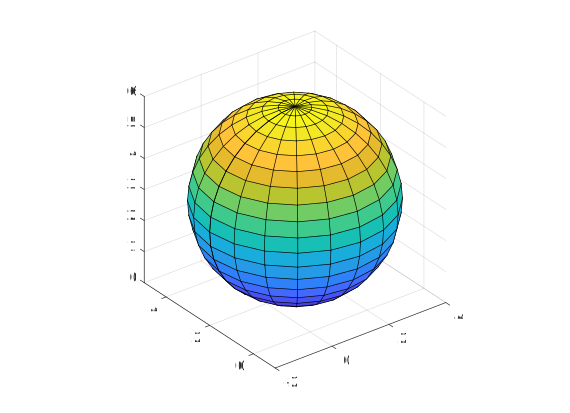Question #5837

# How to write a Matlab function that draw a sphere?

Merged questions

The following Matlab function draws a sphere in the current figure:

``````function [X,Y,Z] = drawSphere(center, radius)
%drawSphere Draw a sphere
%   center are the coordinates of the center [x,y,z]
%   The function returns the points of the sphere

[X,Y,Z] = sphere;
``````drawSphere ([1,2,3],3);